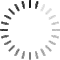# AutoCasting Doubt

0
0

Sir,

if I have piece of code as below:

unsigned char a = -5;

char b = -5;

if(a == b)

print (“characters are same”);

else

print(“characters are different”);

Output is : Characters are different.

How AutoCasting will work in the above case can you please explain?  According to my understanding from your lecture,  when unsigned char and signed char are associated (a == b)  then the unsigned quantity is auto cast  to signed quantity which is higher.  So, I interpret the above association as (( char)a ==  (char) b) ie. (0xFB == 0xFB)  so the answer must be same.

• You must to post comments
0
0

You will get the reason as soon as you print the hexa decimal values as follows:

printf ( “0x%x  ox%x\n”, a, b);

a was assigned 8 bits only and hence value 0xFB.  b is a 32 bit value and gets 0xFFFFFFFB. These are representations for -5 in 8 and 32 bits respectively.

• You must to post comments
Showing 1 result